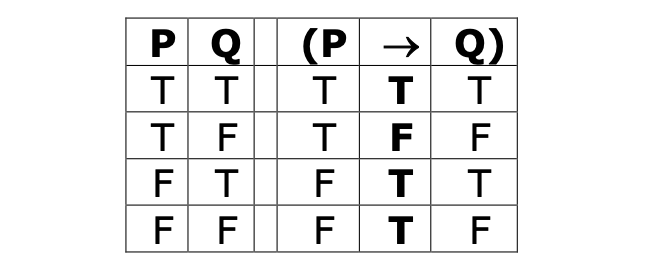## Want to keep learning?

This content is taken from the University of York's online course, Logic: The Language of Truth. Join the course to learn more.
4.3

## University of York# Valid forms of argument involving arrow

Look at the following arguments.

• (P $$\rightarrow$$ Q), P; therefore, Q
• (P $$\rightarrow$$ Q), ~Q; therefore, ~P

Arguments which have these forms are formally valid. We can see this by looking at the truth-table for ‘(P $$\rightarrow$$ Q)’.#### Figure 1. Truth table for the sentence ‘(P $$\rightarrow$$ Q)’

Consider the first argument. It’s valid if and only if there’s no possible situation in which all of the premises are true and the conclusion false. So we can check to see if it’s valid by looking at situations in which all of the premises are true and checking to see if, in any of those, the conclusion is false. If there aren’t any situations like that, then the argument is valid. If there are, it’s not valid.

One of the premises is ‘P’. So, we only need to look at the first two rows at most—those where ‘P’ is true—because only these rows could be counterexamples.

Our other premise is ‘(P $$\rightarrow$$ Q)’. That’s false on the second row, but true on the first.

So, the only kind of situation in which both of the premises are true is that corresponding to the first row of the truth-table. And on that row, ‘Q’ is true. So, in every case in which ‘(P $$\rightarrow$$ Q)’ and ‘P’ are both true, ‘Q’ will be true too. So this argument is formally valid.

Now look at the second argument: (P $$\rightarrow$$ Q), ~Q; therefore, ~P. This is formally valid too. This is, perhaps, a little harder to see, but again we can confirm it by looking at the truth-table for ‘(P $$\rightarrow$$ Q)’.#### Figure 2. Truth table for the sentence ‘(P $$\rightarrow$$ Q)’ (again)

One of the premises is ‘~Q’. That’s only true where ‘Q’ is false; that is, in situations corresponding to the second and fourth rows of the table. Our other premise is ‘(P $$\rightarrow$$ Q)’. That’s false on the second row, but true on the fourth.

So, the only kind of situation in which both of the premises are true is that corresponding to the fourth row of the truth-table. And on that row, ‘P’ is false, so ‘~P’ is true: So, in every case in which ‘(P $$\rightarrow$$ Q)’ and ‘~Q’ are both true, ‘~P’ will be true too. So this argument is formally valid too.